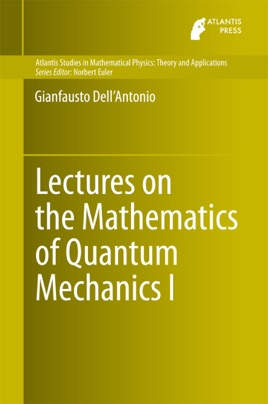• USD 109.99

## Descripción de editorial

The first volume (General Theory) differs from most textbooks as it emphasizes the mathematical structure and mathematical rigor, while being adapted to the teaching the first semester of an advanced course in Quantum Mechanics (the content of the book are the lectures of courses actually delivered.). It differs also from the very few texts in Quantum Mechanics that give emphasis to the mathematical aspects because this book, being written as Lecture Notes, has the structure of lectures delivered in a course, namely introduction of the problem, outline of the relevant points, mathematical tools needed, theorems, proofs. This makes this book particularly useful for self-study and for instructors in the preparation of a second course in Quantum Mechanics (after a first basic course). With some minor additions it can be used also as a basis of a first course in Quantum Mechanics for students in mathematics curricula.

The second part (Selected Topics) are lecture notes of a more advanced course aimed at giving the basic notions necessary to do research in several areas of mathematical physics connected with quantum mechanics, from solid state to singular interactions, many body theory, semi-classical analysis, quantum statistical mechanics. The structure of this book is suitable for a second-semester course, in which the lectures are meant to provide, in addition to theorems and proofs, an overview of a more specific subject and hints to the direction of research. In this respect and for the width of subjects this second volume differs from other monographs on Quantum Mechanics. The second volume can be useful for students who want to have a basic preparation for doing research and for instructors who may want to use it as a basis for the presentation of selected topics.